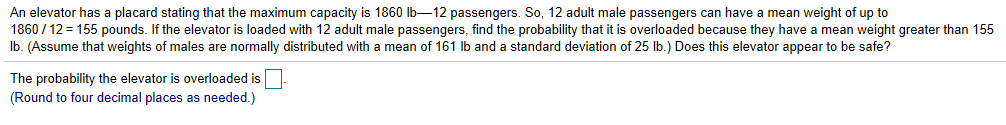# An elevator has a placard stating that the maximum capacity is 1860 lb-12 passengers. So, 12 adult male passengers can have a mean weight of up to1860/12= 155 pounds. If the elevator is loaded with 12 adult male passengers. find the probability that it is overloaded because they have a mean weight greater than 155lb. (Assume that weights of males are normally distributed with a mean of 161 lb and a standard deviation of 25 lb.) Does this elevator appear to be safe?The probability the elevator is overloaded is(Round to four decimal places as needed.)

Question
237 viewshelp_outlineImage TranscriptioncloseAn elevator has a placard stating that the maximum capacity is 1860 lb-12 passengers. So, 12 adult male passengers can have a mean weight of up to 1860/12= 155 pounds. If the elevator is loaded with 12 adult male passengers. find the probability that it is overloaded because they have a mean weight greater than 155 lb. (Assume that weights of males are normally distributed with a mean of 161 lb and a standard deviation of 25 lb.) Does this elevator appear to be safe? The probability the elevator is overloaded is (Round to four decimal places as needed.) fullscreen
check_circle

Step 1

Sampling distribution of sample mean:

The sample mean of a random variable X has a sampling distribution that has population mean or expected value same as that of X and population standard deviation, called the standard error, which is the population standard deviation of X divided by the square root of the sample size n. The parameters are as follows:

Step 2

Distribution of sample mean weight of adult male passengers:

If a variable X is normally distributed with mean, E(Xi) = μ and variance V(Xi) = σ2, then X-bar will also follow normal distribution with mean μ and standard deviation σ/(SQRT(n)).

Step 3

Determine the mean and standard deviation of the sampling distribution of the sample mean weight of adult male passengers:

A random sample of size n = 12 adult male players is selected.

Let X denotes the weight of a randomly selected adult male passenger.

Denote the population mean, that is, the mean weight of all adult male passengers as μ and the standard deviation in weights of all adult male passengers as σ.

Here, μ = 161 lb and σ = 25 lb.

Denote the sample mean weight of 12 randomly s...

### Want to see the full answer?

See Solution

#### Want to see this answer and more?

Solutions are written by subject experts who are available 24/7. Questions are typically answered within 1 hour.*

See Solution
*Response times may vary by subject and question.
Tagged in

### Other